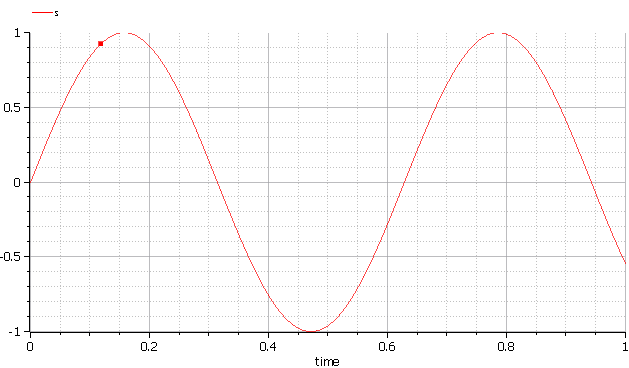# OMMatlab – OpenModelica Matlab Interface¶

OMMatlab – the OpenModelica Matlab API is a free, open source, highly portable Matlab-based interactive session handler for Modelica scripting. It provides the modeler with components for creating a complete Modelica modeling, compilation and simulation environment based on the latest OpenModelica library standard available. OMPython is architectured to combine both the solving strategy and model building. So domain experts (people writing the models) and computational engineers (people writing the solver code) can work on one unified tool that is industrially viable for optimization of Modelica models, while offering a flexible platform for algorithm development and research. OMMatlab is not a standalone package, it depends upon the OpenModelica installation.

OMMatlab is implemented in Matlab and depends on ZeroMQ - high performance asynchronous messaging library and it supports the Modelica Standard Library version 3.2 that is included in starting with OpenModelica 1.9.2.

The Development is in progress and will be soon available.At the moment a prototype of OMMatlab is completed where the users will be able to import the OMMatlab package in Matlab and start to interact with omc through API calls

## Current Prototype¶

The current version of the OMMatlab pacakge contains the following features:

• Import the OMMatlab package in Matlab
• Connect with the OpenModelica compiler through zmq sockets
• Able to interact with the OpenModelica compiler through the available API
• All the API calls are communicated with the help of the sendExpression method implemented in a Matlab pacakge
• The results are returned as strings

## Test Commands¶

To get started, create a OMMatlab session object:

```>>> import OMMatlab.*
>>> omc= OMMatlab()
>>> omc.sendExpression("getVersion()")
'v1.13.0-dev-531-gde26b558a (64-bit)'
'true'
>>> omc.sendExpression("model a Real s; equation s=sin(10*time); end a;")
'{a}'
>>> omc.sendExpression("simulate(a)")
>>> omc.sendExpression("plot(s)")
'true'
```Currently this is an incomplete Beta release. In the final release the OMMatlab interface is planned to implement the more advanced API calls below where the users will have the ability to perform interactive simulation and analysis similar to the enhanced OMPython features.

## List of Planned API support¶

• getQuantities()
• getContinuous()
• getInputs()
• getOutputs()
• getParameters()
• getSimulationOptions()
• getSolutions()
• setInputs()
• setParameters()
• setSimulationOptions()

With the above list of API calls implemented, the users can have more control over the result types, returned using Matlab data structures.